Mathematical and Physical Journal
for High Schools
Issued by the MATFUND Foundation
 Already signed up? New to KöMaL?

# KöMaL Problems in Physics, September 2021

Show/hide problems of signs:## Problems with sign 'M'

Deadline expired on October 15, 2021.

M. 406. At home make an inclined plane of small and variable angle of inclination. Fix the slope at a certain angle of inclination of $\displaystyle \alpha$. Place a cylinder-shaped pencil on the slope such that the symmetry axis of the pencil makes an angle of $\displaystyle \beta$ with a horizontal line lying in the plane of the slope. Investigate, at a certain fixed angle of $\displaystyle \alpha$, at which angle of $\displaystyle \beta$ will the pencil begin to move along the plane such that it

$\displaystyle a)$ slides down, but does not roll at all;

$\displaystyle b)$ rolls down without slipping?

Investigate the rolling and slipping regions, and plot them in a coordinate system of $\displaystyle (\alpha,\beta)$.

(6 pont)

statistics## Problems with sign 'G'

Deadline expired on October 15, 2021.

G. 749. A flight operated by the United Airlines, UA425 passed right in front of the Sun when Andrew McCarthy, a well-known American astrophotographer, took the attached picture. Estimate the distance between the plane and the photographer's camera!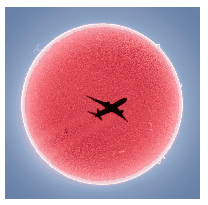(4 pont)

solution (in Hungarian), statistics

G. 750. Jack, whose mass is 35 kg, stands on a bathroom scale. He holds a spring balance, which weighs 0.5 N, in his hand, and he hangs a traditional balance to the spring balance. The weight of the empty traditional balance is 15 N. In one plate of the traditional balance there is a stone, which is balanced by weights whose total mass is 2 kg and 20 dag. What is the reading on the bathroom scale?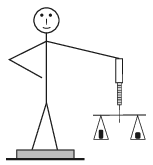(3 pont)

solution (in Hungarian), statistics

G. 751. The image formed by a plane mirror has the same size as the object. However, if we go closer to the mirror we observe ourselves bigger, because the angle of view gets greater. We can see our back by means of two plane mirrors, which are placed approximately opposite and parallel to each other.

Where should we stand in between the two mirrors in order to get the greatest angle of view of our back?

(4 pont)

solution (in Hungarian), statistics

G. 752. The one-ton Mars rover called Perseverance landed successfully on Mars in the middle of February this year. The rover also carried a helicopter drone. What is the weight of the rover on Mars? How could they put the helicopter drone to test on the Earth? Make a suggestion.

(4 pont)

solution (in Hungarian), statistics## Problems with sign 'P'

Deadline expired on October 15, 2021.

P. 5337. Two freight trains are travelling along two parallel railways at a uniform speed. They pass each other in 20 seconds if they move towards each other, while it takes 60 s to pass if they move into the same direction. It takes 40 s for one of the trains and 100 s for the other one to cross a 600-m long bridge.

Determine the speeds and the lengths of the trains.

(4 pont)

solution (in Hungarian), statistics

P. 5338. A pair of dominoes are placed to a third domino as it is shown in the left figure.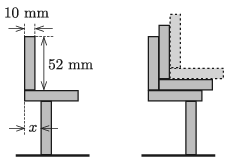$\displaystyle a)$ Determine the possible values of $\displaystyle x$ such that the dominoes are in stable equilibrium.

$\displaystyle b)$ Then several more domino pairs are placed to the dominoes as shown in the right figure. At most how many dominoes can be placed on the domino at the bottom in order that the system remain in equilibrium?

(5 pont)

solution (in Hungarian), statistics

P. 5339. There is a thin rod of length $\displaystyle L=0.6$ m and of negligible mass on a horizontal frictionless surface. Objects of masses $\displaystyle m = 0.2$ kg and $\displaystyle M = 0.8$ kg are attached to the ends of the rod by means of tight threads of negligible mass. The threads are perpendicular to the rod. At a certain moment a force of $\displaystyle F = 8$ N is exerted on the centre of the rod; the force is parallel to the surface and perpendicular to the rod.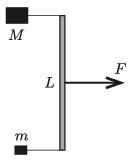$\displaystyle a)$ Determine the acceleration of the centre of the rod at the initial moment.

$\displaystyle b)$ At what point of the rod should the force be exerted in order that the two objects have the same acceleration? What are the tensions in the threads in this case?

(4 pont)

solution (in Hungarian), statistics

P. 5340. One of the great radio telescopes of researchers who search for extraterrestrial life, detected some strange variable-frequency signs, shown in the figure, coming from a distant celestial body. The signs were detected continuously for 45 minutes, and then there was a 45-minute break, then again there were signs for 45 minutes and so on. The middle value of the frequency of the detected signs was $\displaystyle f_0=1.5$ GHz, and the frequency was changing with an amplitude of $\displaystyle \Delta f=40$ kHz, about the middle value $\displaystyle f_0$.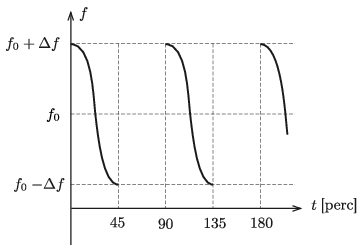The detected radio waves were interpreted by the researchers as signals from an exo-satellite orbiting an exoplanet. It was assumed that the ray joining the Earth and the exoplanet lies in the plane of the orbit of the exo-satellite, and thus the planet mass, radius, and average density could be determined. What values did they get?

(5 pont)

solution (in Hungarian), statistics

P. 5341. A freight train carries a cuboid-shaped container of length $\displaystyle \ell$, width $\displaystyle d$ and height $\displaystyle h$. The container is halfway filled with some liquid of density $\displaystyle \varrho$. What force would the liquid exert on the base and each of the side faces of the container, if the train was able to move at a constant acceleration of $\displaystyle a_0$ for a long time? (The longer edge of the container is parallel to the rails, and the liquid would not flow out of the container even if it was open at its top.)

Data: $\displaystyle \ell=10$ m, $\displaystyle h=d=3$ m, $\displaystyle \varrho=1000~\mathrm{kg/m}^3$, $\displaystyle a_0=1~\mathrm{m/s}^2$.

(5 pont)

solution (in Hungarian), statistics

P. 5342. An object of mass $\displaystyle M$ is hanging of the piston of a vertical fixed cylinder. The cylinder is closed at its top and the mass of the piston is $\displaystyle m$. $\displaystyle Q$ amount of heat is added to the air inside the cylinder, whose initial volume is $\displaystyle V$. The external atmospheric pressure is $\displaystyle p_0$.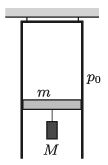$\displaystyle a)$ By what amount does the internal energy of the gas change?

$\displaystyle b)$ How much work is done by the gas? What other energy changes can be related to this work?

(The wall of the cylinder and the piston are made of some thermally insulating material.)

(4 pont)

solution (in Hungarian), statistics

P. 5343. We have three resistors, with resistances 100 ohms, 200 ohms and 300 ohms. The relative uncertainty values of their resistances are 2%, 5% and 10%, respectively. The resistors are connected in series first and then in parallel. What is the equivalent resistance of each connection, and what is the relative uncertainty of each equivalent resistance?

(3 pont)

solution (in Hungarian), statistics

P. 5344. The cross section of a prism is an isosceles triangle with a $\displaystyle 40^\circ$ vertex angle, the refractive index of the material of the prism is 1.6. At what angle of incidence $\displaystyle \alpha$ does a light ray reach one of the (slant) faces of the prism, if this ray travels parallel to the base of the triangle inside the prism? To the other slant face of the prism a piece of glass of refractive index $\displaystyle n$ is placed. What is the value of $\displaystyle n$ if the angle of refraction of the previously described light ray emerging from the prism is $\displaystyle \alpha/2$?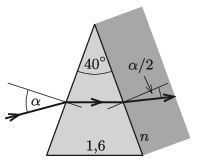(4 pont)

solution (in Hungarian), statistics

P. 5345. Two quarter circles of radius $\displaystyle R$ are formed from two thin tubes and then two incomplete semi-circle shaped tubes are attached to them. The radius of the semicircles is $\displaystyle r$ and the central angle of the missing part is $\displaystyle \alpha$. Then the whole arrangement is attached to a vertical surface as shown in the figure. Then a small marble is dropped, at zero initial speed into the tube at point $\displaystyle A$. The marble slides along the circular arcs of $\displaystyle AB$ and $\displaystyle BC$, then it falls freely between points $\displaystyle C$ and $\displaystyle D$ (oblique projectile motion). Then the marble slides along the circular arcs of $\displaystyle DB$ and $\displaystyle BE$. (Friction and air drag can be neglected everywhere.)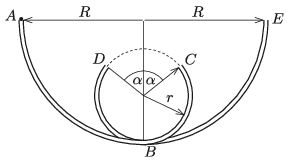$\displaystyle a)$ What is the measure of the angle $\displaystyle \alpha$, if $\displaystyle \frac{R}{r}=\frac{5}{2}$?

$\displaystyle b)$ Investigate at different $\displaystyle \frac{R}{r}$ ratios at which value (or values) of $\displaystyle \alpha$ is it possible for the marble to execute the above described motion.

(6 pont)

solution (in Hungarian), statistics